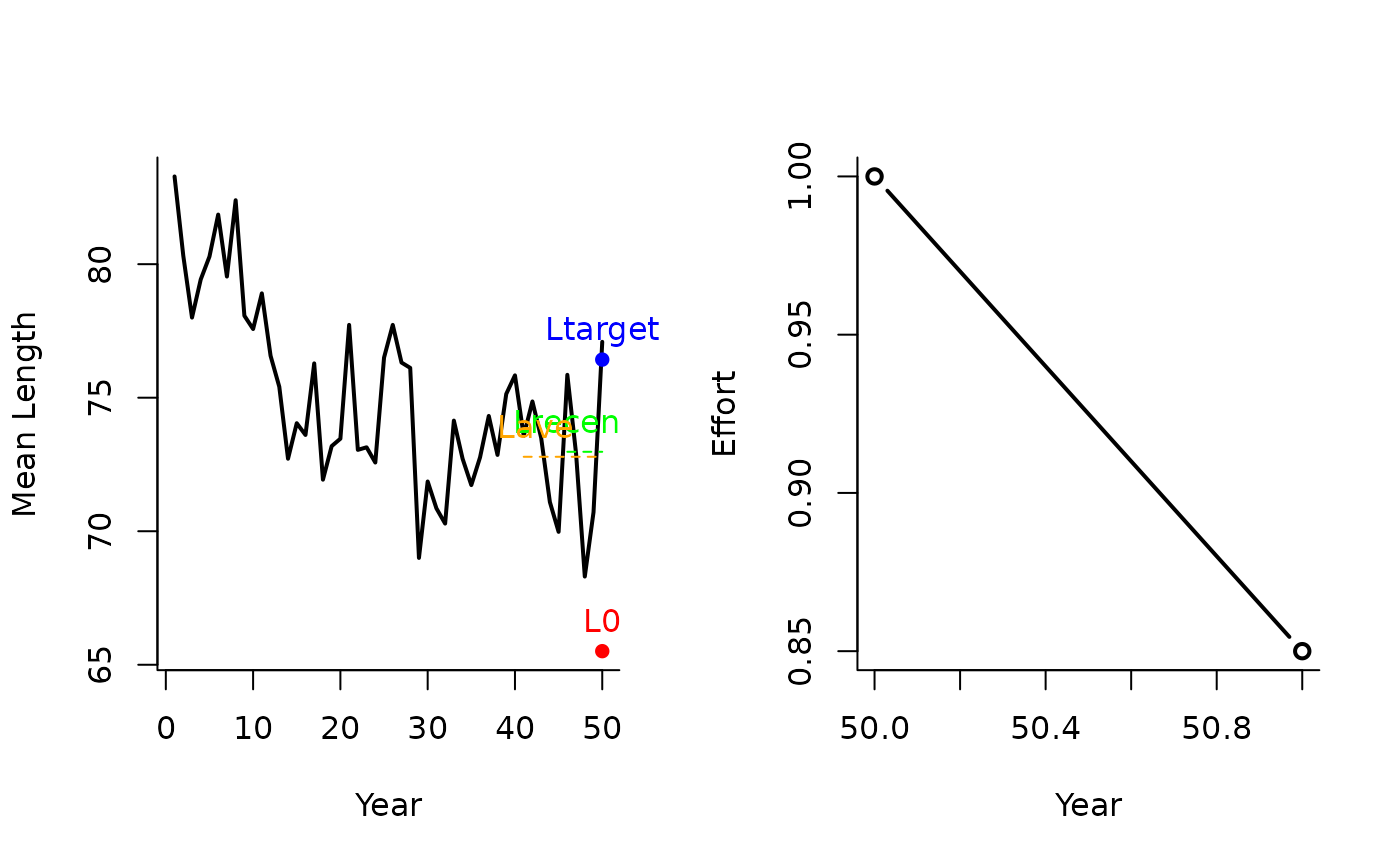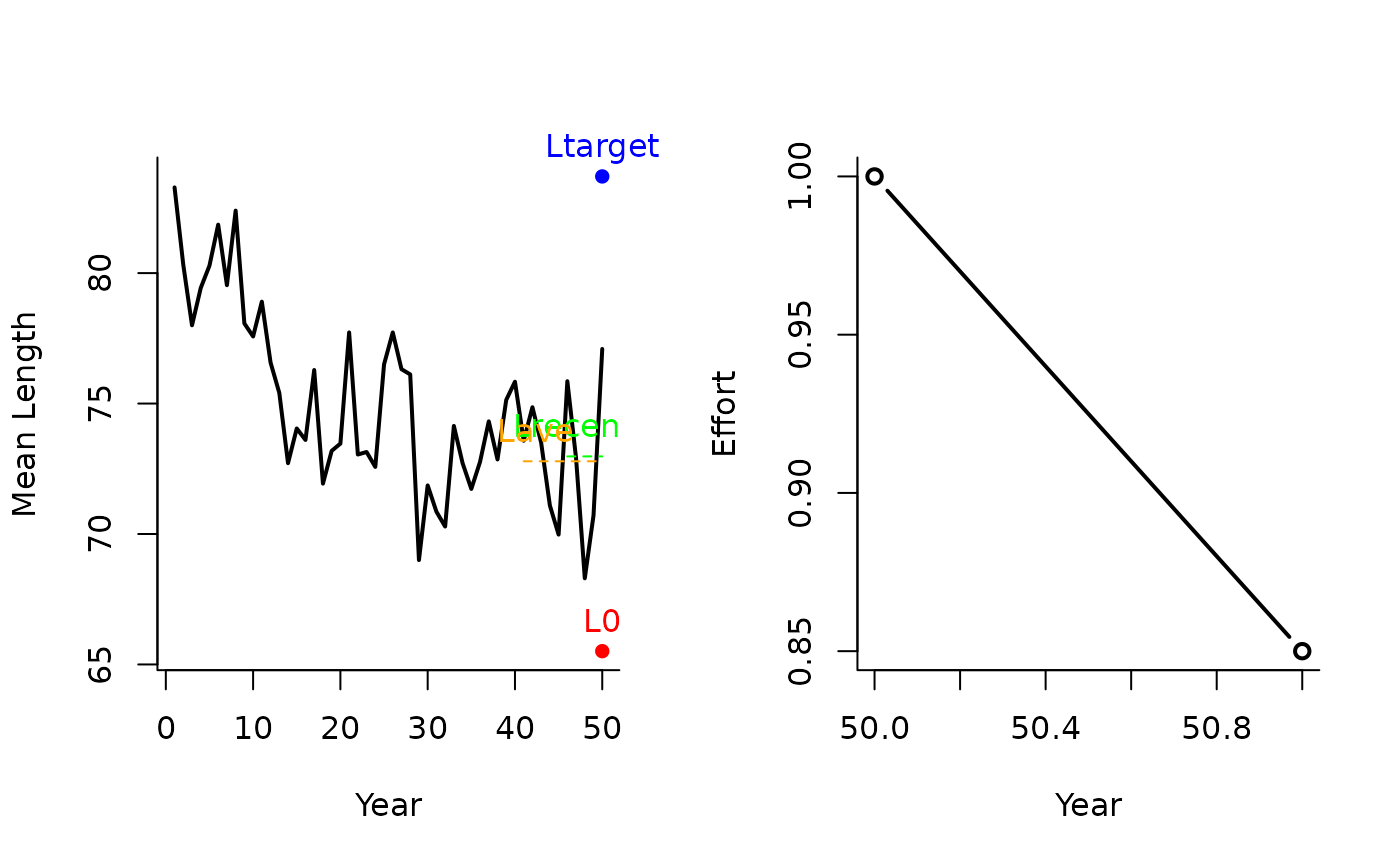A management procedure that incrementally adjusts the TAE to reach a target mean length in catches.

LtargetE1(x, Data, reps = 100, plot = FALSE, yrsmth = 5, xL = 1.05)

LtargetE4(x, Data, reps = 100, plot = FALSE, yrsmth = 5, xL = 1.15)

## Arguments

x

A position in the data object

Data

A data object

reps

The number of stochastic samples of the MP recommendation(s)

plot

Logical. Show the plot?

yrsmth

Years over which to calculate mean length

xL

Parameter controlling the magnitude of the target mean length of catches relative to average length in catches.

## Value

An object of class Rec-class with the TAE slot(s) populated

## Details

Four target length MPs proposed by Geromont and Butterworth 2014. Tested by Carruthers et al. 2015.

The TAE is calculated as:

If $$L_\textrm{recent} \geq L_0$$: $$\textrm{TAE} = 0.5 \textrm{TAE}^* \left[1+\left(\frac{L_\textrm{recent}-L_0}{L_\textrm{target}-L_0}\right)\right]$$

else: $$\textrm{TAE} = 0.5 \textrm{TAE}^* \left[\frac{L_\textrm{recent}}{L_0}^2\right]$$

where $$\textrm{TAE}^*$$ is the effort in the previous year, $$L_\textrm{recent}$$ is mean length in last yrmsth years, $$L_0$$ is (except for L95target) 0.9 average catch in the last 2 x yrsmth historical (pre-projection years) ($$L_\textrm{ave}$$), and $$L_\textrm{target}$$ is (except for L95target) xL $$L_\textrm{ave}$$.

## Functions

• LtargetE1: The least biologically precautionary TAE-based MP.

• LtargetE4: The xL argument is increased to 1.15.

## Required Data

See Data-class for information on the Data object

LtargetE1: LHYear, ML, MPeff, Year

## Rendered Equations

See Online Documentation for correctly rendered equations

Other Length target MPs: Lratio_BHI(), Ltarget1()

T. Carruthers

## Examples

LtargetE1(1, Data=MSEtool::SimulatedData, plot=TRUE)#> Effort
#>   0.85
LtargetE4(1, Data=MSEtool::SimulatedData, plot=TRUE)#> Effort
#>   0.85